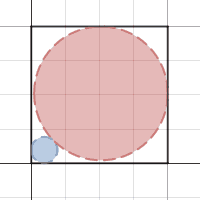# Circle squared

Calculus Level 3Consider a square $ABCD$. Two circles $X$ and $Y$ are drawn such that circle $X$ is tangent to two adjacent sides $AB$ and $AD$, and circle $Y$ is tangent to the other two adjacent sides $CB$ and $CD,$ and circles $X$ and $Y$ are tangent to each other.

As the radius of circle $X$ increases, at what radius of circle $X$ does the sum of the areas of circle $X$ and $Y$ change the slowest?

Express that radius as a fraction of the side of the square $ABCD$, and round to the nearest hundredth.

×

Problem Loading...

Note Loading...

Set Loading...